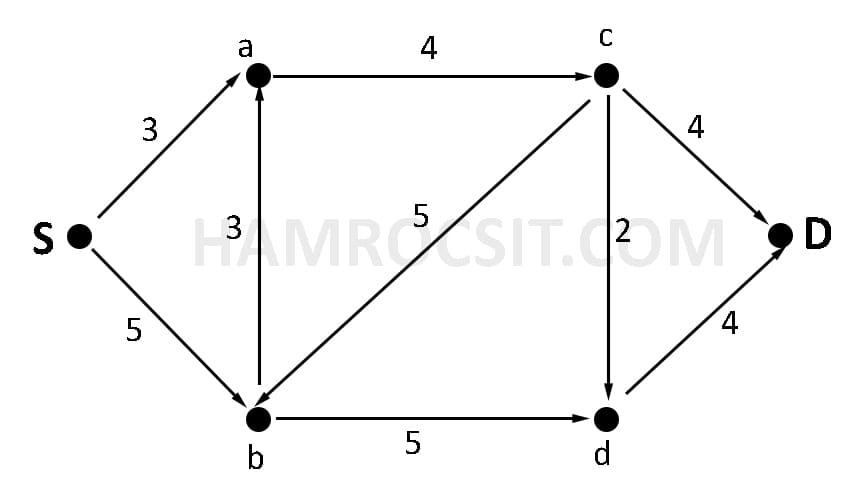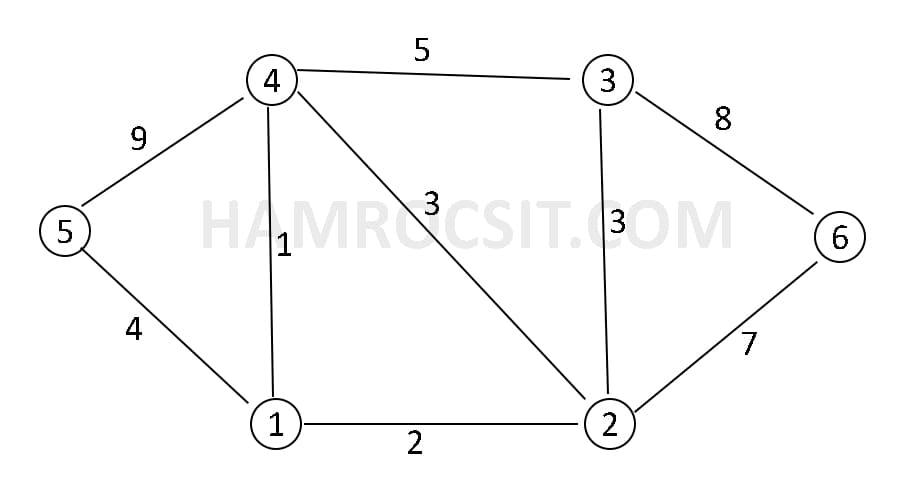### Exam Year

Tribhuvan University

Institute of Science and Technology

2079

Bachelor Level / second-semester / Science

Computer Science and Information Technology( CSC160 )

Discrete Structure

Full Marks: 60 + 20 + 20

Pass Marks: 24 + 8 + 8

Time: 3 Hours

Candidates are required to give their answers in their own words as far as practicable.

The figures in the margin indicate full marks.

Group A

Attemps any TWO questions (2 x 10 = 20)

1

How do you plot the function on graph? Determine whether the function f(x) = x2 is injective, surjective or bijective with reasons. Solve the recurrence relation an = 6an-1 – 9an-2 with initial conditions a0 = 1 and a1 = 6.

2

A group of 8 scientist is composed of 5 chemist and 3 biologist. In how many ways can a committe of 5 be formed that has 3 chemist and 2 biologist? Using mathematical induction prove that 13 + 23 + 33 + ……………….. + n3 = n2(n + 1)2 / 4) for n ≥ 1.

3

Show that the relation R = {(a, b): |a – b| is even} is an equivalence relation in the set of integers. Given the following transport network with the edges labeled with their capacities, find all S-D and their capacities and What is the minimum capacity?Group B

Attemps any EIGHT questions (8 x 5 = 40)

4

List any one example of tautology. Represent the following sentences into predicate logic.

1. Not all employees are loyal
2. All students having good attitude are lovable.
5

Prove that “If the product of two integers a and b are even then either a is even or b is even”, using the contradiction method.

6

Use Chinese Remainder Theorem to find the value of x such that x = 0 ( MOD 2) , x = 2 (MOD 3) and x = 3 (MOD 5).

7

Define bipartile graph with example. State the necessary conditions for the graphs to be isomorphic.

8

State Generalized Pigeonhole Principle. Find the MST from following graph using Kruskal algorithm.9

Given the premises “If it rains or strike holds then the exam will be cancelled. If the doesn’t rain then it will be sunny day. The exam was not cancelled. show that it is sunny day”.

10

Find the value of -2 MOD 3 and 315 MOD 5. Illustrate an example to show the join operation between any two boolean matrixes.

11

Given an example of fallacy. State the necessary and sufficient conditions for a graph to have Euler path and Euler circuit.

12

Find the GCD of 24 and 32 using Extended Euclidean algorithm.